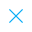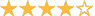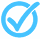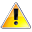Call Support +91-85588-96644

Keep me logged in
You can't leave Captcha Code empty
By submitting this form, you agree to the Terms & Privacy Policy.
ORTests given

ORLinear Equations in One or Two Variables - 2| By TCY

Questions

Time

Highest
score

Level

ENGLISH

Language

Topics Covered:Solving a Pair of Linear EquationsGraphing Linear EquationsArithmeticLinear EquationWord Problems Involving NumbersSolutions of Linear Equations in 2 VariablesLinear EquationsAlgebraic EquationsSolution of System of Linear Equations in 2 VariablesLinear Equation in one VariableThis is a concept-building practice test and may not have exact structure as you would expect in the actual exam. Please exercise your discretion to attempt it or go to structured Featured Section.

Description:
Linear Equation in one Variable - 1
 Type Comment Question
Topper(s) of this Test
•Shruti Bhat

Score: 15/15

•Nishil Faldu

Score: 15/15

•Score: 14/15

•Vansh Mahajan

Score: 14/15

•Sahej Bansal

Score: 14/15Related Tests